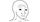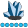somebody
Lapis Lazuli | Level 10

## PROC ARIMA vs PROC TIMESERIES

I am trying to compute the cross-correlation between stock returns and volume. I ran across 3 ways to compute this (PROC ARIMA vs PROC TIMESERIES vs manually computing the correlation). I want to estimate this figure for the whole market, so I compute at the stock level, and then averaging across the market. However, I got 3 different results (although they are quite similar).

I think it is because of how each method deals with missing data. How can I make the 3 the same? what statement am I missing?

``````proc sort data=sample; by stock date;run;
*manual method since using timeseries gives error due to data being too big;
%macro lag();
proc expand data=sample out=stock_sample2;
by stock;
%do i=1 %to 10;
convert volume=volume_lag&i./ transformout=(lag &i.);
%end;
run;
%mend;
%lag;
proc sort data=stock_sample2;by year stock;run;
proc corr data=stock_sample2 outp=cross_corr2 noprint;
by year stock;
var r volume:;
run;
data cross_corr3(rename=(volume=lag)); set cross_corr2;
if substr(_NAME_,1,3) in ("ETF","etf");
if substr(_NAME_,12,3)="lea" then volume=1*substr(_NAME_,16,length(_NAME_)-15);
else if substr(_NAME_,12,3)="lag" then volume=-1*1*substr(_NAME_,15,length(_NAME_)-14);
else volume=0;
keep _NAME_ r volume year stock;
run;
proc sort data=cross_corr3; by year lag;run;
*Average across stocks;
proc means data=cross_corr3 noprint;
by year lag;
output out=cc_manual(drop=_TYPE_ _FREQ_) mean(r)=cc_manual;
run;

*Using PROC ARIMA;
proc sort data=sample; by year stock date;run;
proc arima data=sample; *Estimate the lead/lag correlations;
by year stock;
identify var=volume crosscorr=(r) nlag=10 outcov=cc_arima noprint; * The variable in the crosscorr is the one that gets lagged/led;
run; quit;
proc sort data=cc_arima; by year lag;where CROSSVAR='r';run;
*Average across stocks;
proc means data=cc_arima noprint;
by year lag;
output out=cc_arima_means(drop=_TYPE_ _FREQ_) mean(corr)=cc_arima;
run;

*using PROC TIMESERIES;
proc sort data=sample; by year stock date;run;
proc timeseries data=sample outcrosscorr=crosscor;
var volume r;
by year stock;
id date interval=weekday;
crosscorr lag n ccf/nlag=10;
run;
*Average across stocks;
proc means data=crosscor noprint nway;
var ccf;
class year _name_ _cross_ lag;
output out=cc_ts mean=;
run;
data cc_ts(keep=year lag ccf rename=(ccf=cc_ts));set cc_ts;where _CROSS_='r';run;
*Merge the 3 together;
data cc; merge cc_ts cc_manual cc_arima_means;by year lag;run;``````

1 ACCEPTED SOLUTION

Accepted Solutions

## Re: PROC ARIMA vs PROC TIMESERIES

PROCs ARIMA and TIMESERIES should return the same crosscorrelations when the same values of Y and X are passed to both procedures.  For example, the following code returns identical results between the two procedures--both when Y and X have all nonmissing values, and when Y and/or X have one or more embedded missing values in the DATA= data set passed to the procedures:

``````proc arima data=test;
identify var=y crosscorr=(x) nlag=10 outcov=arima_ccf (where=(crossvar='x')) noprint ;
run;
quit;

proc timeseries data=test outcrosscorr=ts_ccf out=_null_;
var y;
crossvar x;
crosscorr lag n ccov ccf/ nlag=10;
run;``````

(Note that the above code is slightly different than the code you are currently running for these procedures.)

If you observe differences in the results between PROC ARIMA and PROC TIMESERIES, then it could be due to the inclusion of the ID statement in your PROC TIMESERIES step.  If the DATA= data set you are using has gaps in it (such as omitted observations for holidays), then the ID statement in PROC TIMESERIES will first fill in any gaps in the data with missing values prior to computing the crosscorrelations.  The PROC ARIMA code does not do that.  You might want to try either omitting the ID statement in your PROC TIMESERIES step or creating an OUT= data set from your PROC TIMESERIES step and using that data set as input into your PROC ARIMA analysis to see if that resolves the differences you observed.  The following section of the PROC TIMESERIES documentation includes details on how the cross-covariances and crosscorrelations are computed in the TIMESERIES and ARIMA procedures:

The crosscorrelations computed by PROCs ARIMA and TIMESERIES differ from the "manual computation" of the correlations using PROC CORR. When you use PROC CORR to compute the correlation between an analysis variable and the lags of another variable, PROC CORR uses the mean of the analysis variable and the mean of each individual lag variable in the calculations.  On the other hand, PROCs ARIMA and TIMESERIES use the mean of the VAR variable and the mean of the CROSSVAR variable in the calculations--not the mean of each lagged variable.  For example, if you compute Corr(y_t,x_t-4), then PROC CORR uses the mean of Y and the mean of the Lag4(X) variable.  If you examine the crosscorrelation coefficient between Y and X at lag 4 computed by PROC TIMESERIES or PROC ARIMA, then its computation is based on the mean of Y and the mean of X.

The reason crosscovariances and crosscorrelations are computed by time series methods using the overall mean of each variable is because of the assumption of stationarity in both the mean and variance of each series.  This is also noted in the following Wikipedia page under the "Time series analysis" section:

There is no option to change the behavior in either PROC CORR or PROCs TIMESERIES or ARIMA to resolve this discrepancy.  For more details on the computations used in PROC CORR, please see the following documentation link:

I hope this helps!

DW

## Re: PROC ARIMA vs PROC TIMESERIES

PROCs ARIMA and TIMESERIES should return the same crosscorrelations when the same values of Y and X are passed to both procedures.  For example, the following code returns identical results between the two procedures--both when Y and X have all nonmissing values, and when Y and/or X have one or more embedded missing values in the DATA= data set passed to the procedures:

``````proc arima data=test;
identify var=y crosscorr=(x) nlag=10 outcov=arima_ccf (where=(crossvar='x')) noprint ;
run;
quit;

proc timeseries data=test outcrosscorr=ts_ccf out=_null_;
var y;
crossvar x;
crosscorr lag n ccov ccf/ nlag=10;
run;``````

(Note that the above code is slightly different than the code you are currently running for these procedures.)

If you observe differences in the results between PROC ARIMA and PROC TIMESERIES, then it could be due to the inclusion of the ID statement in your PROC TIMESERIES step.  If the DATA= data set you are using has gaps in it (such as omitted observations for holidays), then the ID statement in PROC TIMESERIES will first fill in any gaps in the data with missing values prior to computing the crosscorrelations.  The PROC ARIMA code does not do that.  You might want to try either omitting the ID statement in your PROC TIMESERIES step or creating an OUT= data set from your PROC TIMESERIES step and using that data set as input into your PROC ARIMA analysis to see if that resolves the differences you observed.  The following section of the PROC TIMESERIES documentation includes details on how the cross-covariances and crosscorrelations are computed in the TIMESERIES and ARIMA procedures:

The crosscorrelations computed by PROCs ARIMA and TIMESERIES differ from the "manual computation" of the correlations using PROC CORR. When you use PROC CORR to compute the correlation between an analysis variable and the lags of another variable, PROC CORR uses the mean of the analysis variable and the mean of each individual lag variable in the calculations.  On the other hand, PROCs ARIMA and TIMESERIES use the mean of the VAR variable and the mean of the CROSSVAR variable in the calculations--not the mean of each lagged variable.  For example, if you compute Corr(y_t,x_t-4), then PROC CORR uses the mean of Y and the mean of the Lag4(X) variable.  If you examine the crosscorrelation coefficient between Y and X at lag 4 computed by PROC TIMESERIES or PROC ARIMA, then its computation is based on the mean of Y and the mean of X.

The reason crosscovariances and crosscorrelations are computed by time series methods using the overall mean of each variable is because of the assumption of stationarity in both the mean and variance of each series.  This is also noted in the following Wikipedia page under the "Time series analysis" section:

There is no option to change the behavior in either PROC CORR or PROCs TIMESERIES or ARIMA to resolve this discrepancy.  For more details on the computations used in PROC CORR, please see the following documentation link:

I hope this helps!

DW

Discussion stats